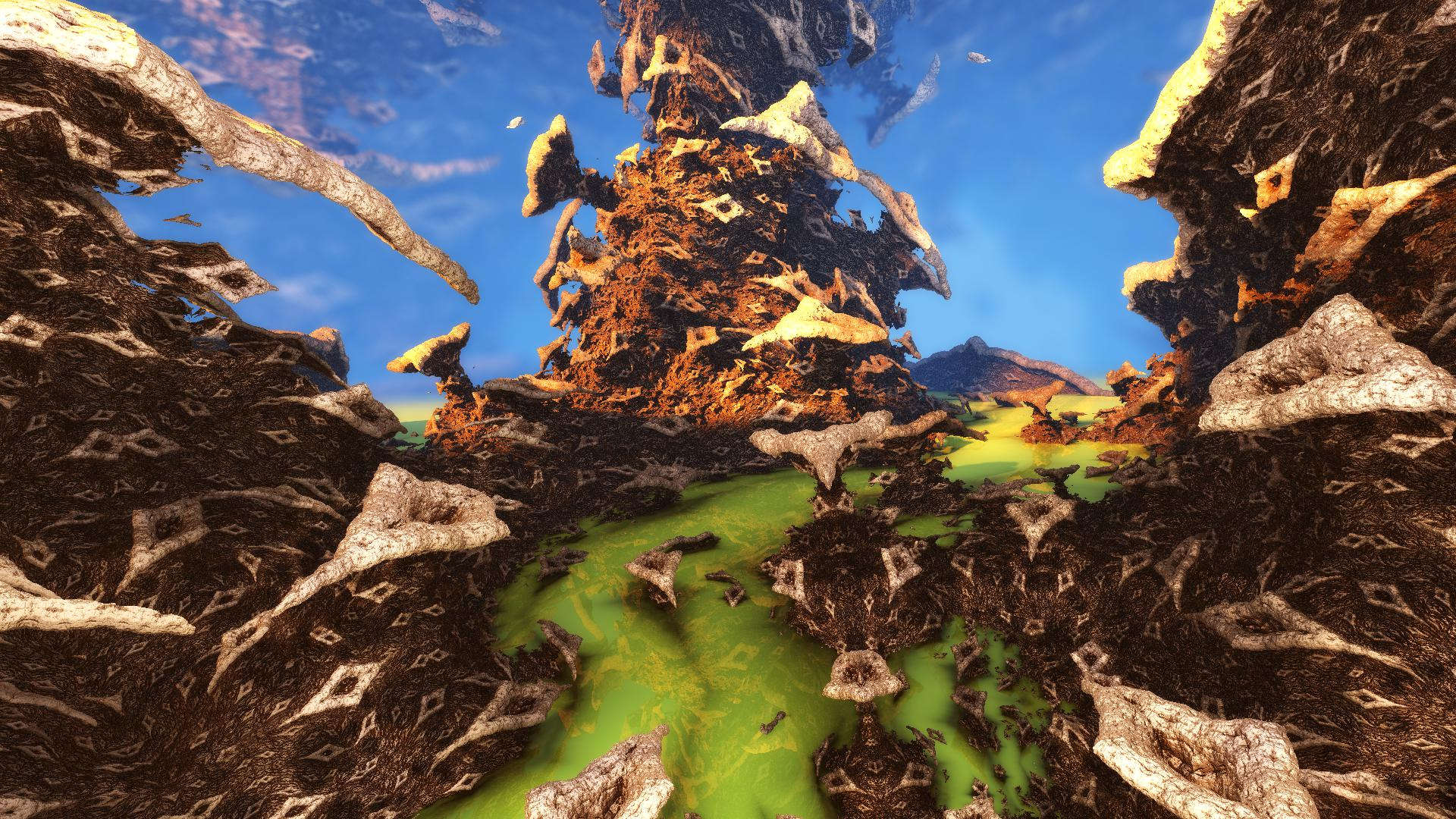News: Check out the originating "3d Mandelbulb" thread here## The All New FractalForums is now in Public Beta Testing! Visit FractalForums.org and check it out!

It's a strange worldPrevious Image | Next Image
Description: Fragmentarium-Asurf DE-Kn8

#info Amazing Surface by Kali - Based on Tglad's Amazing Box
#define providesInit
#include "DE-Kn8.frag"
#define KN_VOLUMETRIC
#define USE_IQ_CLOUDS
#include "MathUtils.frag"
#define USE_INF_NORM
#group AmazingSurface

uniform int Iterations;  slider[0,17,300]
uniform int ColorIterations;  slider[0,3,300]
uniform float Scale;  slider[-3,1.5,3.0]
uniform int FoldType; slider[1,1,3]
uniform vec3 PreTranslation; slider[(-5,-5,-5),(0,0,0),(5,5,5)]
uniform vec2 FoldValues; slider[(0,0),(1,1),(5,5)]
uniform bool Julia; checkbox[false]
uniform vec3 JuliaValues; slider[(-5,-5,-5),(-1,-1,-1),(5,5,5)]
uniform vec3 RotVector; slider[(-1,-1,-1),(1,1,1),(1,1,1)]
uniform float RotAngle; slider[-180,0,180]

mat3 rot;

void init() {
rot = rotationMatrix3(normalize(RotVector), RotAngle);
}

vec4 scale = vec4(Scale, Scale, Scale, abs(Scale))/MinRad2;
float absScalem1 = abs(1-Scale);
float AbsScaleRaisedTo1mIters = pow(abs(Scale), float(1-Iterations));

float DE(vec3 pos) {
vec4 p = vec4(pos,1);
vec3 c = Julia?JuliaValues:p.xyz;
for (int i=0; i<Iterations; i++) {
if (FoldType==1) p.xy=abs(p.xy+FoldValues)-abs(p.xy-FoldValues)-p.xy;
if (FoldType==2) p.xy=FoldValues-abs(abs(p.xy)-FoldValues);
if (FoldType==3) p.xy=abs(p.xy+FoldValues);
p.xyz+=PreTranslation;
float r2 = dot(p.xyz, p.xyz);
if (i<ColorIterations) orbitTrap = min(orbitTrap, abs(vec4(p.xyz,r2)));
p = p*scale;
p.xyz+=c;
p.xyz*=rot;
}
return ((length(p.xyz) - absScalem1) / p.w - AbsScaleRaisedTo1mIters);
}

#preset timemit1
FOV = 0.65625
Eye = -0.967282,1.658636,-0.1424379
Target = 3.104564,-40.57953,0.015433
Up = -0.0475652,-0.0008506,0.9992288
Gamma = 0.8999
ToneMapping = 5
Exposure = 0.833217
Brightness = 0.9803922
Contrast = 0.9470752
Saturation = 0.9733894
GaussianWeight = 1
AntiAliasScale = 0.0995733
Detail = -3.62453
FudgeFactor = 1
Dither = 0.43043
NormalBackStep = 5.957
AO = 0,0,0,1
CamLight = 0.811765,0.909804,0.937255,1.230226
CamLightMin = 0.5628492
Glow = 1,0.721569,0.490196,0.31962
GlowMax = 74
BaseColor = 1,1,1
OrbitStrength = 0.6269285
X = 0.105882,0.129412,0.129412,0.9710345
Y = 0.45098,0.364706,0.219608,1
Z = 0.827451,0.764706,0.772549,0.9241379
R = 0.87451,0.858824,0.67451,0.1235335
BackgroundColor = 0.921569,0.811765,0.168627
CycleColors = true
Cycles = 2.622887
EnableFloor = true NotLocked
FloorNormal = 0.0174703,-0.0356394,-1
FloorHeight = 0.1988835
FloorColor = 0.7254902,1,0.4666667
FocalPlane = 2.54299
Aperture = 0.07814
InFocusAWidth = 1
ApertureNbrSides = 5 NotLocked
ApertureRot = 136.325
ApStarShaped = false NotLocked
ReflectionsNumber = 1 NotLocked
Bloom = true
BloomIntensity = 0.2117982
BloomPow = 1.832865
BloomTaps = 17
DetailAO = -1.544693
MaxRaySteps = 921
MaxDistance = 567.12
AoCorrect = 0.016632
Specular = 0.1102688
SpecularExp = 16
Reflection = 0.2313725,0.172549,0.145098
SpotGlow = true
SpotLight = 1,0.631373,0.109804,8.94961
LightPos = 1.220527,5.686547,1.48405
LightSize = 0.2186207
LightFallOff = 0.289492
LightGlowExp = 0.5777311
HF_Fallof = 2.021722
HF_Const = 0.215917
HF_Intensity = 0.2363636
HF_Dir = -0.3145604,-0.0824176,0.3928571
HF_Offset = -3.393855
HF_Color = 0.4784314,0.6666667,0.8823529,1.118644
HF_Scatter = 1.6635
HF_Anisotropy = 0,0,0
HF_FogIter = 8
CloudScale = 0.57362
CloudFlatness = 0.12432
CloudTops = -7.8968
CloudBase = -8.9474
CloudDensity = 0.09519
CloudRoughness = 0.5894
CloudContrast = 1.7731
CloudColor = 0.905882,0.886275,0.588235
SunLightColor = 0.698039,0.203922,0.105882
Iterations = 84
ColorIterations = 14
Scale = 1.728778
FoldType = 3
PreTranslation = -2.772242,-3.476868,0.13879
FoldValues = 0.6026629,0.6236861
Julia = true
JuliaValues = -4.050633,-5,-0.281294
RotVector = 0.0434783,1,0.3576438
RotAngle = -40.11283
#endpreset

Stats:
Total Favorities: 0 View Who Favorited
Filesize: 542.29kB
Height: 1080 Width: 1920
Discussion Topic: View Topic
Keywords: timemit fragmentarium amazingsurf
Posted by: Tim EmitOctober 14, 2016, 11:53:40 AM

Rating:by 2 members.

 Strange AttractorRating: (None)Filesize: 40.45kBDate: July 01, 2008, 01:30:13 AMComments (0)By: Ross Hilbert Life is StrangeRating:Filesize: 257.18kBDate: April 08, 2010, 10:54:23 AMComments (6)By: AndyAlias Strange AttractorsRating: (None)Filesize: 779.43kBDate: July 31, 2010, 03:39:41 PMComments (0)By: lenord Strange PlanetRating:Filesize: 477.61kBDate: February 06, 2011, 03:50:58 AMComments (1)By: Lee Oliver Strange WatersRating:Filesize: 1.41MBDate: July 01, 2016, 07:28:58 AMComments (1)By: darnoldduck
 Comments (2)3dickulus Global Moderator Fractal SeniorPosts: 1558October 15, 2016, 09:41:59 PMvery primordialSabine Fractal FertilizerPosts: 373October 14, 2016, 07:35:06 PMReally nice, I like the green river and the 'seawater'-dof in particular!